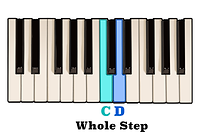top of page

## Interval Identification | Perfect Fifths (5 of 10)

Called Perfect for the same reason as an interval of a Perfect fourth, the ratio of the vibrational frequency of an interval of a Perfect fifth is 3/2. This means that an interval of a Perfect fifth above any given note vibrates at a frequency of one and a half more than the original note.

In other words, if a note vibrates at a frequency of two hundred hertz, or two hundred cycles, per second, then an interval of a Perfect fifth above that note would vibrate at a frequency of three hundred cycles per second.

Put more simply, an interval of a Perfect fifth occurs when any two notes are separated by the distance of seven consecutive half steps. Because two half steps equals one whole step, seven consecutive half steps can also be looked at as being comparatively the same as three whole steps and one half step.

Using this pattern of steps, let's see if we can figure out what an interval of a Perfect fifth above the note C is.Starting on the note C and going up one whole step takes us to the note D.The distance between the note D and the note E is also a whole step.A half step separates the notes E and F.And finally, a whole step above the note F is the note G.

Based on this, an internal of a Perfect fifth above the note C is the note G.In the previous video, we learned that an interval of a Perfect fourth above a given note can also be found on the fourth scale degree of both a corresponding Major and minor scale.

This applies to an interval of a Perfect fifth as well. Looking at the notes of both a C Major and C minor scale we can see that the fifth scale degree in both scales is in fact the note G.When reading an interval of a Perfect fifth on the staff, you might notice that both notes will either be on a space with two lines and one space between them,or on a line with two spaces and one line between them.Memorizing this shape on the staff will make it easier to quickly recognize intervals of a Perfect fifth when reading and writing music. A useful tool for identifying an interval of a Perfect fifth above any given note is the Circle of Fifths.With the Circle of Fifths, each letter progressing in a clockwise direction is an interval of a Perfect fifth above the note that preceded it. In other words, we can see here that the letter at the top of the circle is C. We already know that an interval of a Perfect fifth above the note C is the note G. If we followed the order of steps which produces an interval of a Perfect fifth we would discover that a Perfect fifth above the note G is the note D. An interval of a Perfect fifth above the note D is the note A, and so on.

bottom of page